Jr Math section of MathBitsNotebook.comPolygons (beyond triangles and quadrilaterals**)Topics Standards: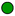NGMS-6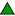CCSS 6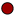NGMS-7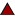CCSS 7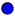NGMS-8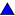CCSS 8

** While polygons include triangles and quadrilaterals, this section will be focusing
primarily on polygons with five, or more, sides.
For work with triangles and quadrilaterals,
see the section Area, Perimeter, Volume.

Polygons (definitions, regular polygons, types)Angles and Polygons (subdivide polygon into triangles to find sum of angles, etc)Area by Decomposing Polygons (also find sum of interior angles, each interior angle)Area by Composing Polygons (into rectangles on grid)Areas of Regular Polygons (discover regular poly area formula, grid area method for both)Surface Area of Polyhedra (composed of polygons beyond triangles and quads)Volume of Polyhedra (composed of polygons beyond triangles and quads)PRACTICE: • Practice with Polygons• Practice with Area of Polygons (decomposition and composition)• Practice with Surface Area and Volume of Polyhedra (figures with polygon bases)• Vocabulary - Word Guess Game (topical vocabulary game - iPad compatible)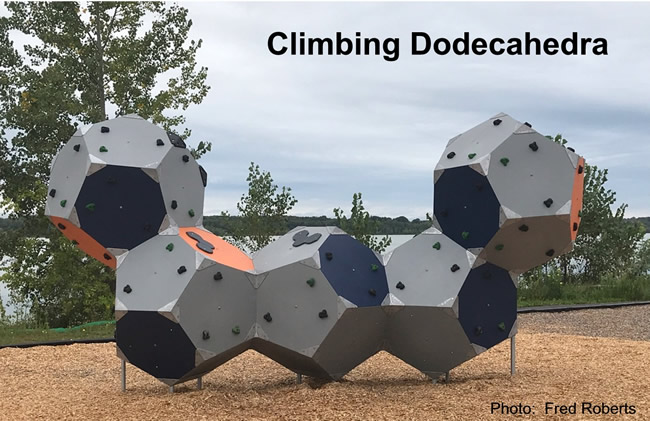Climbing wall in park on Lake Neatahwanta, in Fulton, New York.